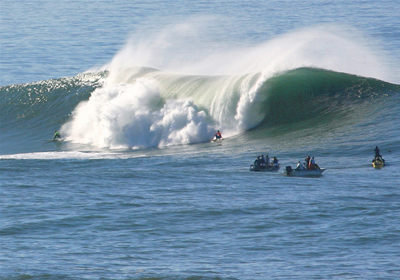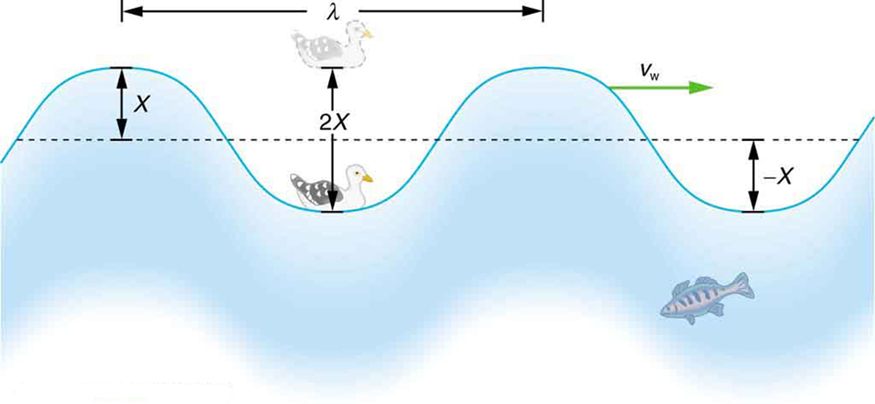# 16.9 Waves

 Page 1 / 7
• State the characteristics of a wave.
• Calculate the velocity of wave propagation.Waves in the ocean behave similarly to all other types of waves. (credit: Steve Jurveston, Flickr)

What do we mean when we say something is a wave? The most intuitive and easiest wave to imagine is the familiar water wave. More precisely, a wave    is a disturbance that propagates, or moves from the place it was created. For water waves, the disturbance is in the surface of the water, perhaps created by a rock thrown into a pond or by a swimmer splashing the surface repeatedly. For sound waves, the disturbance is a change in air pressure, perhaps created by the oscillating cone inside a speaker. For earthquakes, there are several types of disturbances, including disturbance of Earth’s surface and pressure disturbances under the surface. Even radio waves are most easily understood using an analogy with water waves. Visualizing water waves is useful because there is more to it than just a mental image. Water waves exhibit characteristics common to all waves, such as amplitude, period, frequency and energy. All wave characteristics can be described by a small set of underlying principles.

A wave is a disturbance that propagates, or moves from the place it was created. The simplest waves repeat themselves for several cycles and are associated with simple harmonic motion. Let us start by considering the simplified water wave in [link] . The wave is an up and down disturbance of the water surface. It causes a sea gull to move up and down in simple harmonic motion as the wave crests and troughs (peaks and valleys) pass under the bird. The time for one complete up and down motion is the wave’s period $T$ . The wave’s frequency is $f=1/T$ , as usual. The wave itself moves to the right in the figure. This movement of the wave is actually the disturbance moving to the right, not the water itself (or the bird would move to the right). We define wave velocity     ${v}_{\text{w}}$ to be the speed at which the disturbance moves. Wave velocity is sometimes also called the propagation velocity or propagation speed , because the disturbance propagates from one location to another.

Many people think that water waves push water from one direction to another. In fact, the particles of water tend to stay in one location, save for moving up and down due to the energy in the wave. The energy moves forward through the water, but the water stays in one place. If you feel yourself pushed in an ocean, what you feel is the energy of the wave, not a rush of water.An idealized ocean wave passes under a sea gull that bobs up and down in simple harmonic motion. The wave has a wavelength λ size 12{λ} {} , which is the distance between adjacent identical parts of the wave. The up and down disturbance of the surface propagates parallel to the surface at a speed v w size 12{v rSub { size 8{"W"} } } {} .

The water wave in the figure also has a length associated with it, called its wavelength     $\lambda$ , the distance between adjacent identical parts of a wave. ( $\lambda$ is the distance parallel to the direction of propagation.) The speed of propagation ${v}_{\text{w}}$ is the distance the wave travels in a given time, which is one wavelength in the time of one period. In equation form, that is

derivative of first differential equation
why static friction is greater than Kinetic friction
draw magnetic field pattern for two wire carrying current in the same direction
An American traveler in New Zealand carries a transformer to convert New Zealand’s standard 240 V to 120 V so that she can use some small appliances on her trip.
What is the ratio of turns in the primary and secondary coils of her transformer?
nkombo
How electric lines and equipotential surface are mutually perpendicular?
The potential difference between any two points on the surface is zero that implies È.Ŕ=0, Where R is the distance between two different points &E= Electric field intensity. From which we have cos þ =0, where þ is the angle between the directions of field and distance line, as E andR are zero. Thus
sorry..E and R are non zero...
By how much leeway (both percentage and mass) would you have in the selection of the mass of the object in the previous problem if you did not wish the new period to be greater than 2.01 s or less than 1.99 s?
what Is linear momentum
why no diagrams
where
Fayyaz
Myanmar
Pyae
hi
Iroko
hello
Abdu
Describe an experiment to determine short half life
what is science
it's a natural phenomena
Hassan
sap
Emmanuel
please can someone help me with explanations of wave
Benedine
there are seven basic type of wave radio waves, gyamma rays (nuclear energy), microwave,etc you can also search 🔍 on Google :-)
Shravasti
A 20MH coil has a resistance of 50 ohms and us connected in series with a capacitor to a 520MV supply
what is physics
it is the science which we used in our daily life
Sujitha
Physics is the branch of science that deals with the study of matter and the interactions it undergoes with energy
Junior
it is branch of science which deals with study of happening in the human life
AMIT
A 20MH coil has a resistance of 50 ohms and is connected in series with a capacitor to a 250MV supply if the circuit is to resonate at 100KHZ, Determine 1: the capacitance of the capacitor 2: the working voltage of the circuit, given that pie =3.142
Musa
Physics is the branch of science that deals with the study of matter and the interactions it undergoes with energy
Kelly
Heat is transfered by thermal contact but if it is transfered by conduction or radiation, is it possible to reach in thermal equilibrium?
Yes, It is possible by conduction if Surface is Adiabatic
Astronomy
Yeah true ilwith d help of Adiabatic
Kelly
what are the fundamentals qualities
what is physic3
Kalilu
what is physic
Kalilu
Physics? Is a branch of science dealing with matter in relation to energy.
Moses
Physic... Is a purging medicine, which stimulates evacuation of the bowels.
Moses
are you asking for qualities or quantities?
Noman
fundamental quantities are, length , mass, time, current, luminous intensity, amount of substance, thermodynamic temperature.
Shravasti
fundamental quantities are quantities that are independent of others and cannot be define in terms of other quantities there is nothing like Qualities we have only fundamental quantities which includes; length,mass,time, electric current, luminous density, temperature, amount of substance etc
give examples of three dimensional frame of reference
Universe
Noman
Yes the Universe itself
AstronomyByBy Marion CabalfinBy Steve GibbsBy Brooke DelaneyBy Megan EarhartBy Richley CrapoBy Jonathan LongBy Donyea SweetsBy JavaChamp TeamBy OpenStaxBy Stephen Voron